JMSLTM Numerical Library 7.2.0
com.imsl.math

## Class BSpline

• All Implemented Interfaces:
Serializable, Cloneable
Direct Known Subclasses:
BsInterpolate, BsLeastSquares

```public abstract class BSpline
extends Object
implements Serializable, Cloneable```
BSpline represents and evaluates univariate B-splines.

B-splines provide a particularly convenient and suitable basis for a given class of smooth ppoly functions. Such a class is specified by giving its breakpoint sequence, its order k, and the required smoothness across each of the interior breakpoints. The corresponding B-spline basis is specified by giving its knot sequence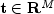. The specification rule is as follows: If the class is to have all derivatives up to and including the j-th derivative continuous across the interior breakpoint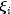, then the numbershould occur k - j - 1 times in the knot sequence. Assuming that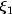and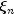are the endpoints of the interval of interest, choose the first k knots equal toand the last k knots equal to. This can be done because the B-splines are defined to be right continuous nearand left continuous near.

When the above construction is completed, a knot sequenceof length M is generated, and there are m: = M-k B-splines of order k, for example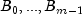, spanning the ppoly functions on the interval with the indicated smoothness. That is, each ppoly function in this class has a unique representation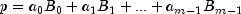as a linear combination of B-splines. A B-spline is a particularly compact piecewise polynomial function.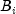is a nonnegative function that is nonzero only on the interval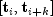. More precisely, the support of the i-th B-spline is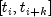. No piecewise polynomial function in the same class (other than the zero function) has smaller support (i.e., vanishes on more intervals) than a B-spline. This makes B-splines particularly attractive basis functions since the influence of any particular B-spline coefficient extends only over a few intervals.

BsInterpolate.html Example, BsLeastSquares.html Example, Serialized Form
• ### Field Summary

Fields
Modifier and Type Field and Description
`protected double[]` `coef`
The B-spline coefficient array.
`protected double[]` `knot`
The knot array of length n + order, where n is the number of coefficients in the B-spline.
`protected int` `order`
Order of the spline.
• ### Constructor Summary

Constructors
Constructor and Description
`BSpline()`
• ### Method Summary

Methods
Modifier and Type Method and Description
`double` `derivative(double x)`
Returns the value of the first derivative of the B-spline at a point.
`double[]` ```derivative(double[] x, int ideriv)```
Returns the value of the derivative of the B-spline at each point of an array.
`double` ```derivative(double x, int ideriv)```
Returns the value of the derivative of the B-spline at a point.
`double[]` `getKnots()`
Returns a copy of the knot sequence.
`Spline` `getSpline()`
Returns a `Spline` representation of the B-spline.
`double` ```integral(double a, double b)```
Returns the value of an integral of the B-spline.
`double` `value(double x)`
Returns the value of the B-spline at a point.
`double[]` `value(double[] x)`
Returns the value of the B-spline at each point of an array.
• ### Methods inherited from class java.lang.Object

`clone, equals, finalize, getClass, hashCode, notify, notifyAll, toString, wait, wait, wait`
• ### Field Detail

• #### coef

`protected double[] coef`
The B-spline coefficient array.
• #### knot

`protected double[] knot`
The knot array of length n + order, where n is the number of coefficients in the B-spline.
• #### order

`protected int order`
Order of the spline.
• ### Constructor Detail

• #### BSpline

`public BSpline()`
• ### Method Detail

• #### derivative

`public double derivative(double x)`
Returns the value of the first derivative of the B-spline at a point.
Parameters:
`x` - a `double` specifying a point at which the derivative is to be evaluated
Returns:
a `double` containing the value of the first derivative of the B-spline at the point `x`
• #### derivative

```public double[] derivative(double[] x,
int ideriv)```
Returns the value of the derivative of the B-spline at each point of an array.
Parameters:
`x` - a `double` array of points at which the derivative is to be evaluated
`ideriv` - an `int` specifying the derivative to be computed. If zero, the function value is returned. If one, the first derivative is returned, etc.
Returns:
a `double` array containing the value of the derivative the B-spline at each point of the array `x`
• #### derivative

```public double derivative(double x,
int ideriv)```
Returns the value of the derivative of the B-spline at a point.
Parameters:
`x` - a `double` specifying a point at which the derivative is to be evaluated
`ideriv` - an `int` specifying the derivative to be computed. If zero, the function value is returned. If one, the first derivative is returned, etc.
Returns:
a `double` containing the value of the derivative of the B-spline at the point `x`
• #### getKnots

`public double[] getKnots()`
Returns a copy of the knot sequence.
Returns:
a `double` array containing a copy of the knot sequence.
• #### getSpline

`public Spline getSpline()`
Returns a `Spline` representation of the B-spline.
Returns:
a `Spline` representation of the `BSpline`
• #### integral

```public double integral(double a,
double b)```
Returns the value of an integral of the B-spline.
Parameters:
`a` - a `double` specifying the lower limit of integration
`b` - a `double` specifying the upper limit of integration
Returns:
a `double` which specifies the B-spline integral value from `a` to `b`
• #### value

`public double value(double x)`
Returns the value of the B-spline at a point.
Parameters:
`x` - a `double` specifying the point at which the B-spline is to be evaluated
Returns:
a `double` giving the value of the B-spline at the point x
• #### value

`public double[] value(double[] x)`
Returns the value of the B-spline at each point of an array.
Parameters:
`x` - a `double` array of points at which the B-spline is to be evaluated
Returns:
a `double` array containing the value of the B-spline at each point of the array `x`
JMSLTM Numerical Library 7.2.0